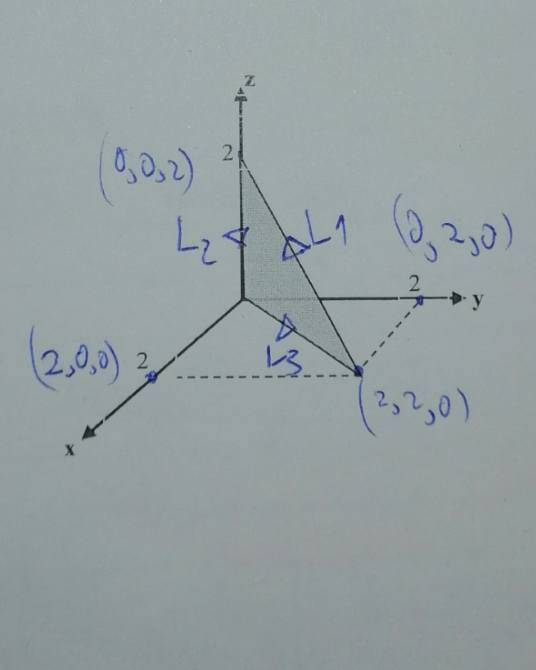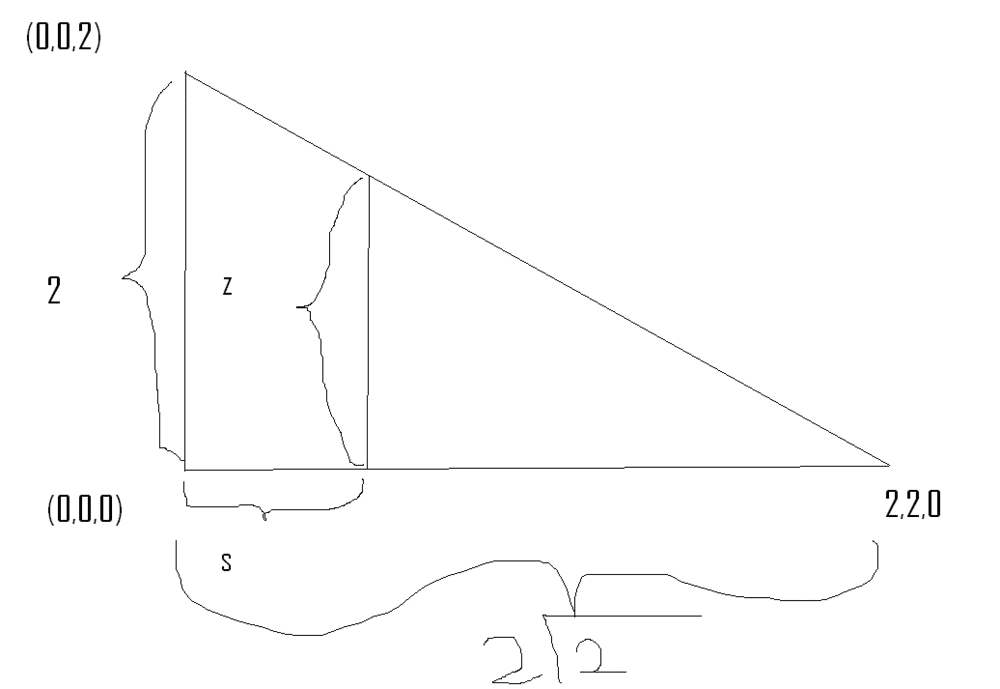# Applying Stoke's theorem on an s,φ,z vector when the given shape is in x,y,z

satanikoskioftes
Homework Statement:
Test stokes theorem on v(s,φ,z)=(ssinφ)S-2zΦ+(2z-ssinφ)Ζ
My given surface has those points as x,y,z coordinates (0.0.2) (0.0.0) (2.2.0)
Its basically a triangle that one side is on the z axis, the other one is on the xy (x=y). The rotation is counterclockwise

Test stokes theorem on v(s,φ,z)=s(2+sinφ)S-(ssinφcosφ)Φ+3zΖ
My given surface has those points as x,y,z coordinates (0.0.3) (2.0.3) (2.2.3)
So its another triangle

capital letter are vectors
Relevant Equations:
∫(∇×F)da=∮F⋅dl
To be honest i don't know from where to start. I know how i can test the stokes theorem if i have a cylindrical shape and a cylindrical vector or spherical vector and a spherical shape but here I am out of ideals.
The first thing i tried was to compute the left part of the stokes theorem but i don't know how to compute the da part
My second attempt was to covert my cylindrical coordinates to x,y,z. If i do that i get some pretty weird integrals though and i don't think that this is the smartest way.
So i think my two main problems are:
1) How to find the da
2) How to express my xyz lines of the triangles to cylindrical moves and use them for my fdl parts

timetraveller123
did you try drawing out the triangles they said if you did then you can what will be the da the triangles are nice enough to have simple da

for both the triangles the dl of two of the three legs of the triangle only involve differential of one coordinate the other leg's dl can be composed of two coordinate differential. you can then relate the two involved differentials to simplify into one integral . for example the first problem
one of legs is just dz the other one -ds the other one is two integrals of dz and ds. ds and dz can be related to each other.
i only tried the first one and it checked out.thanks

satanikoskioftes
Thanks for the reply. Can you analyse what simple da means? Because to me on this level nothing is simple :P
Of course i drew the triangles so let's go to the problems one by one.
In the first problem:
1) My first dl is super easy since only the z axis has "movement" from 2 to 0. x=y=0 so i only have dz
2) On the second dl I am moving from 0 to π/4 (if I am correct). z=0 and s=2*root(2) so i only have dφ here (is this right though or am i missing something?)
3) I have no idea what to do on the third one because my s changes as the z changes but i cannot find the relationship between the two
4) As for the da imi staying on cylindrical coordinates? If so what do i do? How do i determine the da and the points of integration?
In the second problem:
1) Again first dl looks super easy but...im going from x=2 to x=0, z=3 and y=0. Can i assume that φ=0, ζ=3 and is going from 0 to 2 so i only have ds?
2) Now things get weird for me. I see that i only have dy. So i tried to make a relation with x=scosφ since x=2 while y moves. this gives 2=scosφ. Now there are two ways. Either cosφ=2/s --> sinφdφ=(2/s^2)ds or s=2/cosφ --> ds=[-2/(cosφ)^2]sinφdφ. Which of those is right and what do i use as points of integration (if I am on the correct path here)?
3) Here it looks like I am moving on the x=y line from 2root(2) to 0. If this is the case can i just go z=3 φ=π/4 and and s moves from 2root(2) to 0? Or am i missing something (im a bit confused because in the first dl i had an s=2 but now i have a bigger s but i haven't used any ds. Except if this happens and the second dl)?
4) Again no idea how to get da and points of integration.

timetraveller123
hi
problem 1)
1)
yes this is correct only dz is involved although if i am not wrong i think you have to (0,0,0) to (0,0,2) rather than other way around since by the right hand rule your loop must give the area element . i am not too sure about this you might just get a negative value if you go the other way round i think
2)
i am not exactly sure which path you mean here
but my path 2 was from (0,0,2) to (2,2,0)
in this path the phi doesn't change only z and s changes. you have to relate dz and ds to simplify to one integral
3)
here your dl becomes -ds as you go from (2,2,0) to (0,0,0) like going on circle radius to origin so your dl is -ds minus because your going opposite to how it is defined
4)
and for area element all points on this triangle have the same area element which simplies the area integral if you look at the triangle (0,0,0)(0,0,2)(2,2,0) which unit vector do you know is perpendicular to this triangle at all points that is the area element

for problem 2)
i haven't done it yet i am a little bit busy now i will get back to you but i think is same it has a constant da two of the paths only have one differential the hard one is from (2,0,3) to (2,2,3) where phi and s changes with z constant so you have to relate d phi and ds after which you can do the line integral.

thanks

satanikoskioftesHere is the drawing. First dl z goes from 2 to 0. Second dl s goes from 0 to 2root(2) third one is the L1 line but i don't have a clue on how to relate s to z and what i could use as points of integration. Now for the da i think that only Z is perpendicular. So i will have dxdyZ? I only have a x=y line left. How do i translate this to cylindrical and how imi getting my points of integration?
If i guessed correctly you must took the opposite path

Homework Helper
2022 Award
What is $\varphi$ if $$\frac yx = \tan\varphi = 1$$ and $x$ and $y$ are positive?

timetraveller123
but i don't have a clue on how to relate s to z
have you tried similar triangles to relate them

Now for the da i think that only Z is perpendicular
the da has to be perpendicular to the triangle at every point which unit vector
can you think of which is like this how will this triangle look like if you see it from z axis you shouldn't be able to see the height of triangle you only see a line what unit vector will be perpendicular to that line

So i will have dxdyZ
not quite i think it might be easier to work in cylindrical

I only have a x=y line left. How do i translate this to cylindrical and how imi getting my points of integration?
do you know why in line L2 if you do it in cylindrical there is no integral over phi but only over ds and dz then how can you relate them using similar triangles

If i guessed correctly you must took the opposite path
yes
thanks

satanikoskioftes
Oh i think i see it now. The only one perpendicular to triangle is φ right? So i would have to have something like da = dsdzΦ right? Now i somehow have to find the points of integration (any tips on that?)
Also what do you mean by similar triangles? I am not familiar with this technique. I can get all the angels of the triangle by computing the L1L2L3 and then cos(οf the angle that I am looking). Also i can do l2^2 which is z plus l3^2 which is s = l1^2 but this doesn't seem to help me or I am still not getting it

Last edited:
timetraveller123
The only one perpendicular to triangle is φ right? So i would have to have something like da = dsdzΦ right?
yes that's what i meant

Also what do you mean by similar triangles?
it is one way to relate ds and dz for the path l1 or you can use the angles you computed to relate z and s and thus dz and ds
how about for any random point on path l1 how would relate z and s?

satanikoskioftes
I found z/s = root(3)/3 so dz= [root(3)/3]ds. So now i guess that if this is correct i can integrate either from z 0 -->2 or s 0 -->2root(3)

timetraveller123
I found z/s = root(3)/3 so dz= [root(3)/3]ds. So now i guess that if this is correct i can integrate either from z 0 -->2 or s 0 -->2root(3)
not quite right but how did you get ## \frac{z}{s} = \frac{\sqrt{3}}{3}##
and s goes from ##0## to ##2 \sqrt{2}##

Last edited:
satanikoskioftes
I said that if z is l2 and s is l1, then l1=root( (2-0)^2 + (2-0)^2 + (0-2)^2) = 2root(3). And then the cos(οf l1l2) = root(3)/3. I feel a bit dumb now. It must be something so simple and for some weird reason I am not getting it

timetraveller123
nah you are just being a little careless
yes
##
L_1 = 2\sqrt{3}
##
but this is not s

when you go from point (2,2,0) to (0,0,0)
your s changes from what to what

satanikoskioftes
from 2root(2) to 0. But I am trying to find a relation with L1 and now I am computing L3

timetraveller123
yes

lets say you are halfway up L1 what is s and z of that point

satanikoskioftes
one and root2 i guess?

timetraveller123
yes

now relate this s and zsatanikoskioftes
tan in the 2.2.0 point = z/s. I don't know how i missed that. I really thank you for your help. I love the fact that you don't give a simple answer but you guide me to the answer

•timetraveller123
timetraveller123
wait no
tan in 2.2.0 point is ##\frac{z}{2\sqrt{2} -s}##

•satanikoskioftes
satanikoskioftes
Ye my bad. The reason why its 2root(2)-s and not s-2root(2) is due to the fact that when z goes 2 the s goes 0?

timetraveller123
it is just because s is smaller than 2 root 2